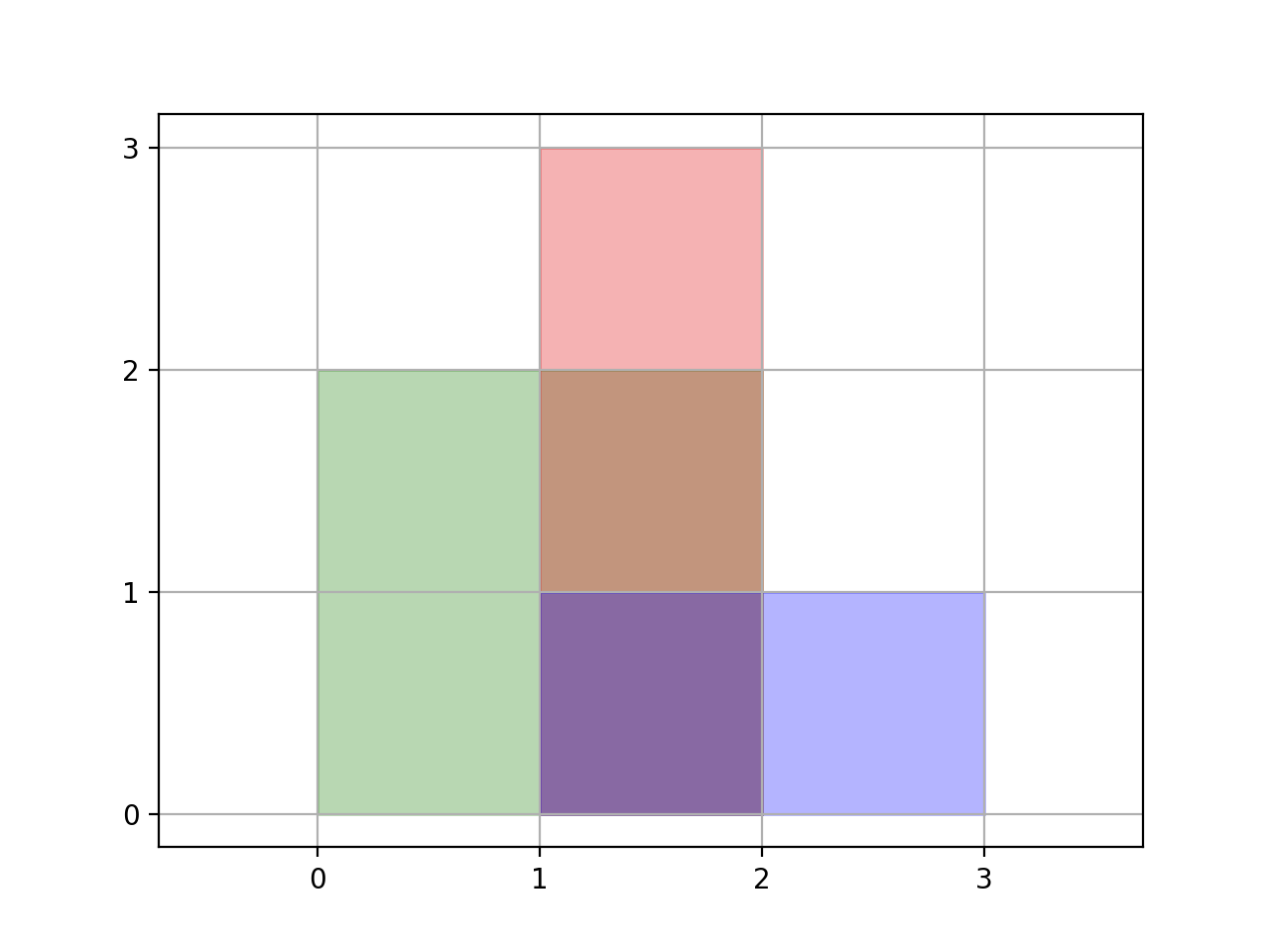# GeetCode Hub

We are given a list of (axis-aligned) `rectangles`. Each `rectangle[i] = [xi1, yi1, xi2, yi2] `, where `(xi1, yi1)` are the coordinates of the bottom-left corner, and `(xi2, yi2)` are the coordinates of the top-right corner of the `ith` rectangle.

Find the total area covered by all `rectangles` in the plane. Since the answer may be too large, return it modulo `109 + 7`.

Example 1:```Input: rectangles = [[0,0,2,2],[1,0,2,3],[1,0,3,1]]
Output: 6
Explanation: As illustrated in the picture.
```

Example 2:

```Input: rectangles = [[0,0,1000000000,1000000000]]
Output: 49
Explanation: The answer is 1018 modulo (109 + 7), which is (109)2 = (-7)2 = 49.
```

Constraints:

• `1 <= rectangles.length <= 200`
• `rectanges[i].length = 4`
• `0 <= rectangles[i][j] <= 109`
• The total area covered by all rectangles will never exceed `263 - 1` and thus will fit in a 64-bit signed integer.

class Solution { public int rectangleArea(int[][] rectangles) { } }